#### Matrices and Systems of Equations

Definition of Matrix: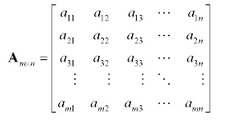a) It is the rectangular array of real numbers.

b) Consists of m rows by n columns.

c) Named by using the capital letters.

d) First subscript is the row and second subscript is the column.

Terminology:

a) It is a matrix with m rows and n columns and is termed as a matrix of order m x n.

b) The square matrix is a matrix with an equivalent number of rows and columns. As the number of rows and columns are similar, it is state to have order n.

c) The main diagonal of a square matrix are the elements from upper left to the lower right of matrix.

d) The row matrix is a matrix which consists of only one row.

e) The column matrix is a matrix which consists of only one column.

f) The matrix with just one row or one column is termed as a vector.

Converting Systems of Linear Equations to Matrices:

Each and every equation in the system becomes a row. Each and every variable in the system becomes a column. Variables are dropped and the coefficients are positioned to a matrix. When the right hand side is comprised, it is termed as an augmented matrix. When the right hand side is not comprised, it is termed as a coefficient matrix.

The system of linear equations:

x + y - z = 1
3x - 2y + z = 3
4x + y - 2z = 9

It becomes the augmented matrix: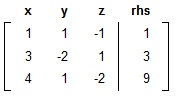Elementary Row Operations:

Elementary Row Operations are the operations which can be executed on a matrix which will generate a row-equivalent matrix. When the matrix is an augmented matrix, constructed from the system of linear equations, then the row-equivalent matrix will contain similar solution set as the original matrix.

Whenever working with systems of linear equations, there were three operations you could execute which would not modify the solution set.

a) Swap two equations.

b) Multiply the equation by a non-zero constant.

c) Multiply the equation by a non-zero constant and add it to the other equation, by replacing that equation.

Whenever a system of linear equations is transformed to an augmented matrix, each and every equation becomes a row. Therefore, there are now three elementary row operations that will generate a row-equivalent matrix.

a) Swap two rows.

b) Multiply a row by a non-zero constant.

c) Multiply a row by the non-zero constant and add it to the other row, by replacing that row.

Row-Echelon and Reduced Row-Echelon Forms:

These are the Row-equivalent forms of a matrix. One can simply solve a system of linear equations whenever matrices are in one of such forms.

Row-Echelon Form:

The matrix is in row-echelon form when the given conditions are met.

a) When there is a row of all zeros, then it is at bottom of the matrix.

b) The first non-zero element of any row is one. That element is termed as the leading one.

c) The leading one of any row is to the right of leading one of the preceding row.

Note:

a) The leading one of a row doesn’t have to be to the instant right of the leading one of previous row.

b) The matrix in row-echelon form will contain zeros beneath the leading ones.

c) Gaussian Elimination places a matrix to row-echelon form, and then back substitution is needed to finish finding the solutions to system.

d) The row-echelon form of a matrix is not essentially exclusive.

Reduced Row-Echelon Form
:

The matrix is in reduced row-echelon form whenever all the conditions of row-echelon form are met and all the elements above, and also below, the leading ones are zero.

a) When there is a row of all zeros, then it is at bottom of the matrix.

b) The first non-zero element of any row is one. That element is termed as the leading one.

c) Leading one of any row is to the right of leading one of the preceding row.

d) All the elements above and beneath a leading one are zero.

Note:

a) Leading one of a row doesn’t have to be to the instant right of leading one of the preceding row.

b) The matrix in row-echelon form will contain zeros of both above and beneath the leading ones.

c) Gauss-Jordan Elimination puts a matrix to reduce the row-echelon form.

d) No back substitution is needed to finish the solutions to the system.

e) The reduced row-echelon form of a matrix is exclusive.

Gaussian Elimination:

a) Write down a system of the linear equations as an augmented matrix.

b) Execute the elementary row operations to place the matrix to row-echelon form.

c) Transform the matrix back to a system of the linear equations.

d) Use back substitution to get all the answers.

Gauss-Jordan Elimination:

a) Write down a system of linear equations as the augmented matrix.

b) Execute the elementary row operations to place the matrix to the reduced row-echelon form.

c) Transform the matrix back to a system of the linear equations.

d) No back substitution is essential.

Pivoting:

a) Pivoting is a procedure that automates the row operations essential to put a matrix to row-echelon or reduced row-echelon form.

b) In specific, pivoting makes the elements above or below the leading one to zeros.

Types of Solutions:

There are three kinds of solutions that are possible whenever solving a system of the linear equations:

Independent:

a) Consistent.

b) Unique Solution.

c) A row-reduced matrix consists of similar number of non-zero rows as variables.

d) The left hand side is generally the identity matrix, however not essentially.

e) There should be at least as many equations as the variables to obtain an independent solution.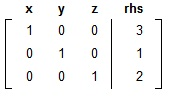Whenever you transform the augmented matrix back to the equation form, you get x = 3, y = 1, and z = 2.

Dependent:

a) Consistent.

b) Many solutions.

c) Write the answer in parametric form.

d) The row-reduced matrix consists of more variables than non-zero rows.

e) There does not have to be a row of zeros, however there generally is.

f) This could as well happen when there is less equation than the variables.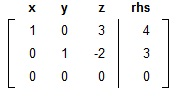The first equation will be x + 3z = 4. Solving for x provides: x = 4 - 3z.

The second equation will be y - 2z = 3. Solving for y provides y = 3 + 2z.

The z column is not cleared out (that is, all the zeros except for one number) therefore the other variables will be stated in terms of z. Thus, z will be the parameter t and the solution is:

x = 4 - 3t, y = 3 + 2t, z = t

Inconsistent:

a) No Solution.

b) It is a row-reduced matrix consists of a row of zeros on the left side; however the right hand side is not zero.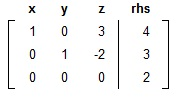There is no solution here. We can write as null set Ø, the empty set {}, or no solution.

Latest technology based Algebra Online Tutoring Assistance

Tutors, at the www.tutorsglobe.com, take pledge to provide full satisfaction and assurance in Algebra help via online tutoring. Students are getting 100% satisfaction by online tutors across the globe. Here you can get homework help for Algebra, project ideas and tutorials. We provide email based Algebra help. You can join us to ask queries 24x7 with live, experienced and qualified online tutors specialized in Algebra. Through Online Tutoring, you would be able to complete your homework or assignments at your home. Tutors at the TutorsGlobe are committed to provide the best quality online tutoring assistance for Algebra Homework help and assignment help services. They use their experience, as they have solved thousands of the Algebra assignments, which may help you to solve your complex issues of Algebra. TutorsGlobe assure for the best quality compliance to your homework. Compromise with quality is not in our dictionary. If we feel that we are not able to provide the homework help as per the deadline or given instruction by the student, we refund the money of the student without any delay.